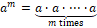## Weekly Overview

Weekly Topics

The focus of this week’s instruction is to deepen students’ understanding of:

• Writing and Evaluating Expressions – Addition and Subtraction
• Writing and Evaluating Expressions – Multiplication and Division
• Writing and Evaluating Expressions – Exponents
• One-Step Equations – Addition and Subtraction
• One-Step Equations – Multiplication and Division

Materials Needed

• Pencil and Paper
• Index Cards
• Individual Whiteboards

Standard(s) Covered

6.EE.A.2 Write, read, and evaluate expressions in which variables stand for numbers.

a.   Write expressions that record operations with numbers and with variables. For example, express the calculation "Subtract y from 5" as 5 - y.

c.   Evaluate expressions at specific values of their variables. Include expressions that arise from formulas used in real-world problems. Perform arithmetic operations, including those involving whole number exponents, in the conventional order when there are no parentheses to specify a particular order (Order of Operations).

6.EE.B.7 Solve real-world and mathematical problems by writing and solving one step equations of the form x + p = q and px = q for cases in which p, q, and x are all nonnegative rational numbers.

Representations• Equation (An equation is a statement of equality between two expressions.)
• Equivalent Expressions (Two expressions are equivalent if both expressions evaluate to the same number for every substitution of numbers into all the variables in both expressions.)
• Exponential Notation for Whole Number Exponents (Let m be a nonzero whole number. For any number a, the expression am is the product of m factors of a (i.e.,).
• Expression (An expression is a numerical expression, or it is the result of replacing some (or all) of the numbers in a numerical expression with variables.)
• Solution of an Equation (A solution to an equation with one variable is a number such that the number sentence resulting from substituting the number for all instances of the variable in both expressions is a true number sentence.  If an equation has more than one variable, then a solution is an ordered tuple of numbers such that the number sentence resulting from substituting each number from the tuple into all instances of its corresponding variable is a true number sentence.)
• Variable (A variable is a symbol (such as a letter) that is a placeholder for a number.)

Familiar Terms

• Distribute
• Expand
• Product
• Properties of Operations (distributive, commutative, associative)
• Quotient
• Sum
• Term
• Variable or Unknown Number